## Example Questions

2 Next →

### Example Question #11 : How To Find The Equation Of A Perpendicular Line

Linefollows the equation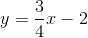.

State the equation, in slope-intercept form, of line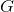, which is perpendicular to lineand intersects it at point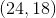.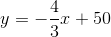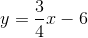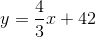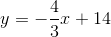Explanation:

Since linefollows the equation, we can surmise its slope is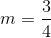. Thus, it follows that any line perpendicular towill have a slope of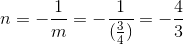.

Since we also know at least one point on line, we can use point slope form to find an initial equation for our line.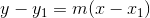---->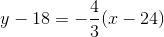.

Next, we can simplify to reach point-slope form.---->.

Thus, linein slope-intercept form is.

### Example Question #91 : Coordinate Geometry

Which of the following equations represents a line that goes through the pointand is perpendicular to the line?Explanation:

In order to solve this problem, we need first to transform the equation from standard form to slope-intercept form:Transform the original equation to find its slope.First, subtractfrom both sides of the equation.Simplify and rearrange.Next, divide both sides of the equation by 6.The slope of our first line is equal to. Perpendicular lines have slopes that are opposite reciprocals of each other; therefore, if the slope of one is x, then the slope of the other is equal to the following:Let's calculate the opposite reciprocal of our slope:The slope of our line is equal to 2. We now have the following partial equation:We are missing the y-intercept,. Substitute the x- and y-values in the given pointto solve for the missing y-intercept.Add 4 to both sides of the equation.Substitute this value into our partial equation to construct the equation of our line:2 Next →

### All ACT Math Resources